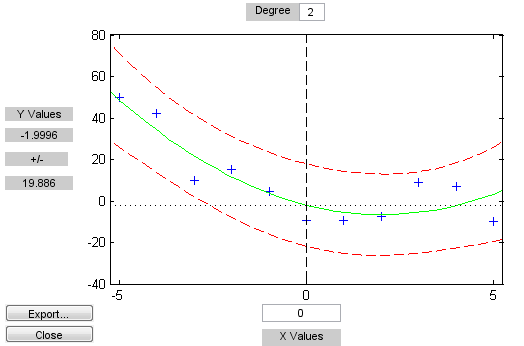# polytool

Interactive polynomial fitting

## Syntax

```polytool(x,y) polytool(x,y,n) polytool(x,y,n,alpha) polytool(x,y,n,alpha,xname,yname) h = polytool(...) ```

## Description

`polytool(x,y)` fits a line to the vectors `x` and `y` and displays an interactive plot of the result in a graphical interface. You can use the interface to explore the effects of changing the parameters of the fit and to export fit results to the workspace.

`polytool(x,y,n)` initially fits a polynomial of degree `n`. The default is `1`, which produces a linear fit.

`polytool(x,y,n,alpha)` initially plots `100(1 - alpha)`% confidence intervals on the predicted values. The default is `0.05` which results in 95% confidence intervals.

`polytool(x,y,n,alpha,xname,yname)` labels the `x` and `y` values on the graphical interface using `xname` and `yname`. Specify `n` and `alpha` as `[]` to use their default values.

`h = polytool(...)` outputs a vector of handles, `h`, to the line objects in the plot. The handles are returned in the degree: data, fit, lower bounds, upper bounds.

## Examples

### Interactive polynomial fitting

This example shows how to start an interactive fitting session with `polytool`.

```rng('default') % for reproducibility x = -5:5; y = x.^2 - 5*x - 3 + 5*randn(size(x));```

Fit a quadratic (degree-2) model with `0.90` confidence intervals.

```n = 2; alpha = 0.1; polytool(x,y,n,alpha)```## Version History

Introduced before R2006a